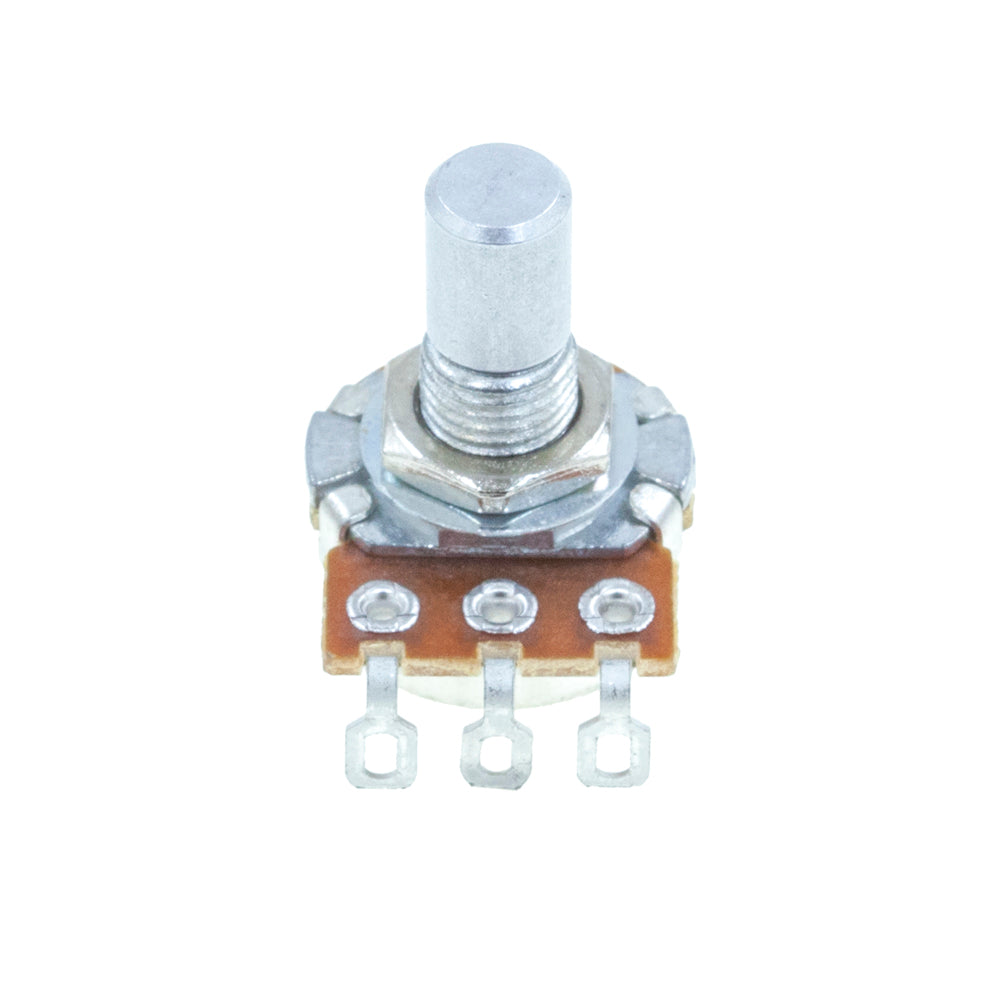### My Cart

Close# Mammoth or Alpha PotentiometerReverse Log (C)16mm Single GangSolder Lug

\$0.55

What does the "K" stand for?
250,000 ohms = 250 Kilo ohms = 0.250 Mega ohms
250,000 Ω = 250 KΩ = 0.250 MΩ
250,000 E = 250 K = 0.250 M

The standard measurement of resistance is ohms, which is symbolized either with the Greek letter Omega, Ω, or by the letter "E." stands for kilo, which means 1000 or alternatively and simply written as 10^3. So the on potentiometers represents 1000 ohms. You will also run across M.

M is the equivalent of 1000 kilos or 1,000,000 ohms, and can also be written as 10^6.

Potentiometer A, B, & C -- What is the difference?

The letter before the value corresponds to the taper of the potentiometer. The taper is the relationship between the rotation of the potentiometer's shaft to the changing resistance.

A - Audio Taper, also know as Logarithmic, or sometimes shortened to Log

An audio taper potentiometer increases slowly at first and then increases more quickly the further you turn it. An example of the relationship between an audio taper potentiometer's rotation and resistance, starting from the furthest left position and rotating towards the right, would be: a 1/4 turn would be ~ 5% of the total resistance, a 1/2 turn would be ~ 10% of the total, and then the rest of the rotation would be a rapid increase towards the total resistance of the potentiometer. To the human ear in order to hear twice the volume there needs to be an increase of ten times. This type of potentiometer is designed to match the human ears' logarithmic nature of hearing volume and provide a smoother transition from low volume to high volume when compared to a linear taper.

B - Linear Taper

A linear taper potentiometer increases in a linear manner as you would expect (i.e., 1, 2, 3, 4) as you rotate the potentiometer to the right. This means if we start with the potentiometer in the utmost left hand position and rotate it rightward we would get a relationship as follows: a 1/4 turn is equivalent to a 1/4 of the total resistance, a 1/2 turn is equal to 1/2 the total resistance, and a full turn is equal to the total amount of resistance.

C- Reverse Audio Taper

A reverse audio taper potentiometer also increases logarithmically, but backwards compared to a regular audio taper. The resistance increases very quickly on this type of potentiometer until you reach the halfway point, from which it increases very slowly. A 1/4 turn on the potentiometer would be ~ 75% of the total resistance, a 1/2 of turn would ~ 95% of the total resistance, and a full turn would be the total resistance.

W-

This is special type of potentiometer used for the tone circuit in Tube Screamer overdrives.